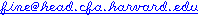May 2002

# Are smaller wheels faster?

This debate doesn't come up often in "regular bike" lists because wheelsizes are relatively fixed, but on recumbent mailing lists, this is a hot topic, with typical wheels ranging from 16 to 27 inches.

From a pure physics point of view, is one better than the other? First of all, it's important to understand that the rear wheel size is one of the gear ratios in the drivetrain. A smaller rear wheel is compensated for by bigger gearing in the rest of the drivetrain, but for the same overall gear ratio, you'll get the same ratio of force between the pedal and the road regardless of wheelsize.

But perhaps there are deeper issues in simple physics? Some wonder if smaller wheels have less inertia and therefore give greater acceleration, while others believe that the smaller wheel has to spin faster, so it is a wash. But when you do the math, smaller wheels win.

The kinetic energy in the wheel is a measure of how much energy you've spent just getting the wheel to spin rather than investing that energy into the road. Here's the equation for the kinetic energy of a rotating object:

```  r is radius
m is mass
I is rotational inertia
E is energy
w is angular velocity
P is the constant Pi

E = 1/2 I w2

rotational inertia is obtained by this equation (for hollow cylinders):

I = 1/2 m r2

but w is radians per second, which is rotations per second (rps) times radians per rotation, which is 2 P

w = 2 P rps

rotations per second (rps) is velocity/circumference
circumference is 2 P r
so rps = v/(2 P r) therefore:

w = 2 P (v/(2 P r)) = v/r

This gives us an energy equation of:
E = 1/2 I (v2/r2)

Now we work on the I term, which as stated above is based on mass and radius.
But of course mass depends on radius.  The mass of a cylinder is:
m = density 2 P r
Let's let the constant C be "density 2 P":
m = C r
So:

I = C r r2 = C r3

and then

E = 1/2 C r3 (v2/r2)

collapse the 1/2 into the constant, and combine terms, and we get:

E = C r v2
```

### Conclusion

This means that for any given forward speed of the bicycle (v), the energy required just to get the wheel up to speed is greater for larger radius wheels. And this relationship is linear, e.g. a wheel that is 50% larger (in radius) will require 50% more energy to reach the same speed (note that this is only wheel rotation energy, not the much larger amount of energy needed to accelerate the bike and rider).

So this issue is finally settled, right? Unfortunately not. It takes this much energy to get the wheel up to speed, but once it's there, you only need to add more energy to make up for what has been lost to friction, and here the larger wheel wins.

Larger wheels have less rolling resistance, for several reasons. First, they won't drop (as much) into a smaller hole as a smaller wheel would. Second, They have greater leverage for lifting the wheel over bumps. Third, there is less deformation of the tire at the contact patch on the ground. Fourth, smaller wheels require faster chain speeds, which will have higher frictional losses. Fifth, for similar reasons they will have higher hub friction.

It's also possible that they have more wind resistance due to the higher spinning speeds, although it's more likely that the lower profile is more significant and that they have less wind resistance. Certainly for fully-faired recumbents, smaller wheels allow for a lower body profile.

But losses due to mechanical friction within the drivetrain on top quality bikes are actually very low. Rolling resistance on the road however can be more significant. So how much difference does the lower inertia of smaller wheels really make?

A 200 pound (90.9 kg) bike/rider combination moving at 20 mph (8.94 m/s) has a kinetic energy of 3632 joules. Additionally you have rims/tires/tape/spokes that weigh around 800 grams, which at this speed (and 27inch wheels) will need about 15 joules per wheel (note that the cogs and freewheel add very little to the rotational inertia because though they are heavy, they have radiuses much smaller than the rim). So about 1 percent of the energy you expend while accelerating to 20 mph goes into the rotation of the wheels. A 13.5 inch wheel would give you roughly a half of a percent improvement in accelaration (actually even less, as these calculations ignore the energy you lost during acceleration to wind resistance and rolling resistance).

I don't have good numbers right now on rolling resistance differences between different wheel sizes that are similarly inflated (emailif you have good data), but my gut reaction is that you lose more in rolling resistance than you gain in accelaration.

With such small differences, smaller wheels might make sense for the very short standing sprints on tracks. But in most applications the superior comfort and rolling resistance of larger wheels wins. Even here though, the advantage is probably not huge. If your application is well-suited to smaller wheels (e.g. a recumbent or a folding bicycle) you don't need to worry about the difference.

• My Cycling Page
 My Home Professional Home TomSaraZac Home Email Work Email